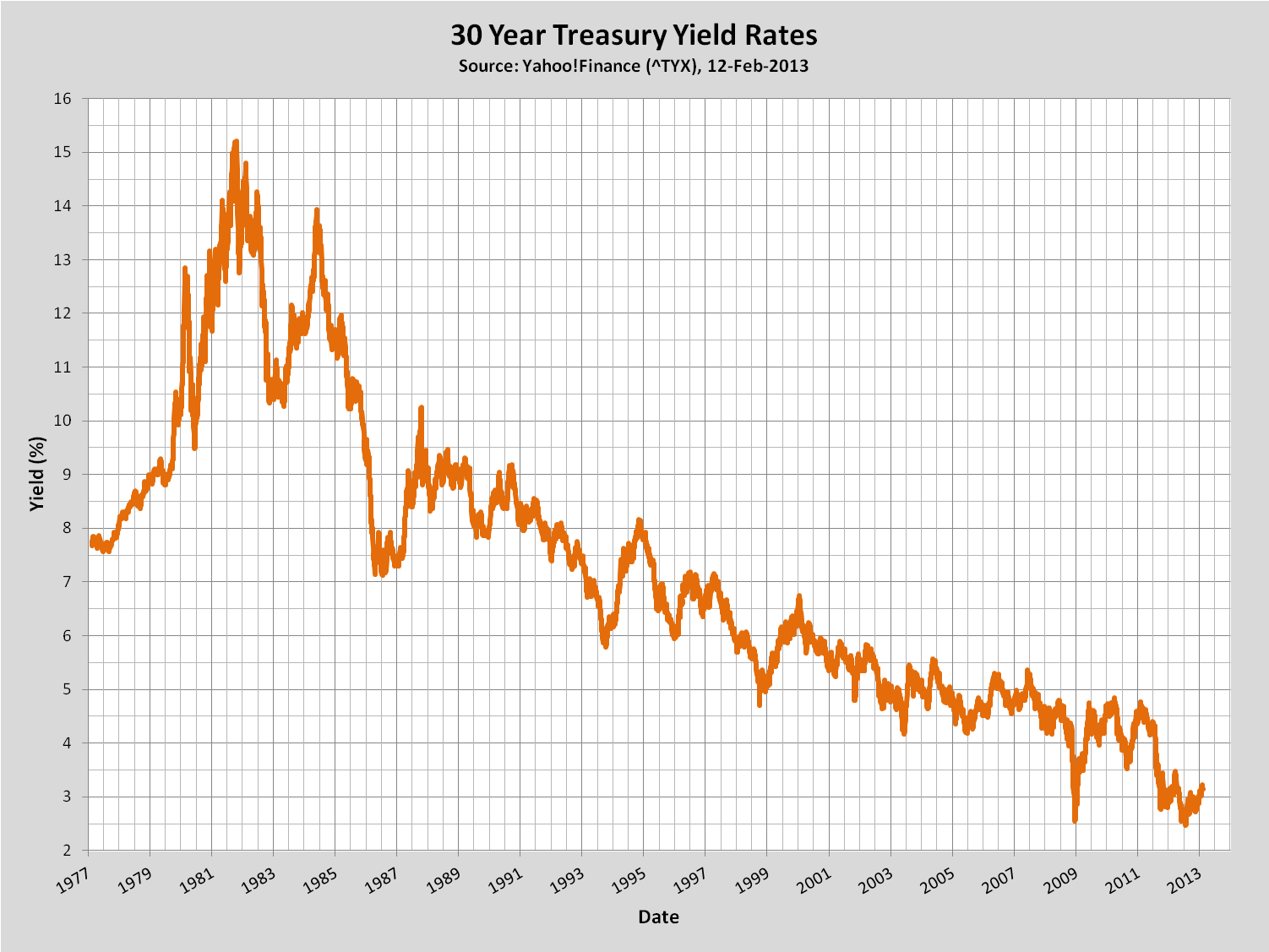# Mortgage Math

## Wonkish Details

Jump to the Results section directly if you're not interested in the theory.

#### Amortization formulas

The formulas for amortization are as follows (see here for detailed derivation). If we have a mortage with principal $P$, monthly interest rate $r$, and term $n$, then the monthly payment, $A$, is given by $$A = P\left[{r\left(1+r\right)^n\over \left(1+r\right)^n-1}\right]\tag{2}$$ The interest accumulated in the $m^{th}$ period (and, consequently, the interest portion of the $m^{th}$ payment) is $$I_m = r p_{m-1}, \tag{4}$$ which is the tax-deductible portion.

#### Inflation, Long-Term Bond Yields, and the Discount Rate

Now we factor in the discount rate. This is essentially the time-value of money, and is usually a function of some essentially risk-free investment (e.g., 30-year Treasury Bonds) and your own personal pet variables such as the rate of inflation.

See below for a plots of historical data of inflation rates and long-term yields (click on a figure to see a larger version).## Results

There are two things of interest
• Is it worth paying points and closing costs? What's the break-even point taking taxes, etc., into account
• Should you prepay? To calculate the NPV you have to make some assumptions about discount rate, so there's some judgment involved. But you can do "what-if".
 Marginal Tax Rate Max. Discount Rate Term   Scenario A Principal Yearly Interest Rate Points Closing Costs %   %   years     $% %$Figure 1. Monthly PaymentsFigure 2. NPV of Cumulative PaymentsScenario B Principal Yearly Interest Rate Points Closing Costs $% %$Figure 3. Break-even analysis of the two scenarios
Notes:
• Only equal-term loans are compared. To compare different terms (e.g., 15-year and 30-year) run the calculations separately. A future version may include comparison of different terms, but Figur 3 doesn't make sense in this case.
• Assume that closing costs are tax-deductible. Non-deductible closing costs will be included in a future rev.